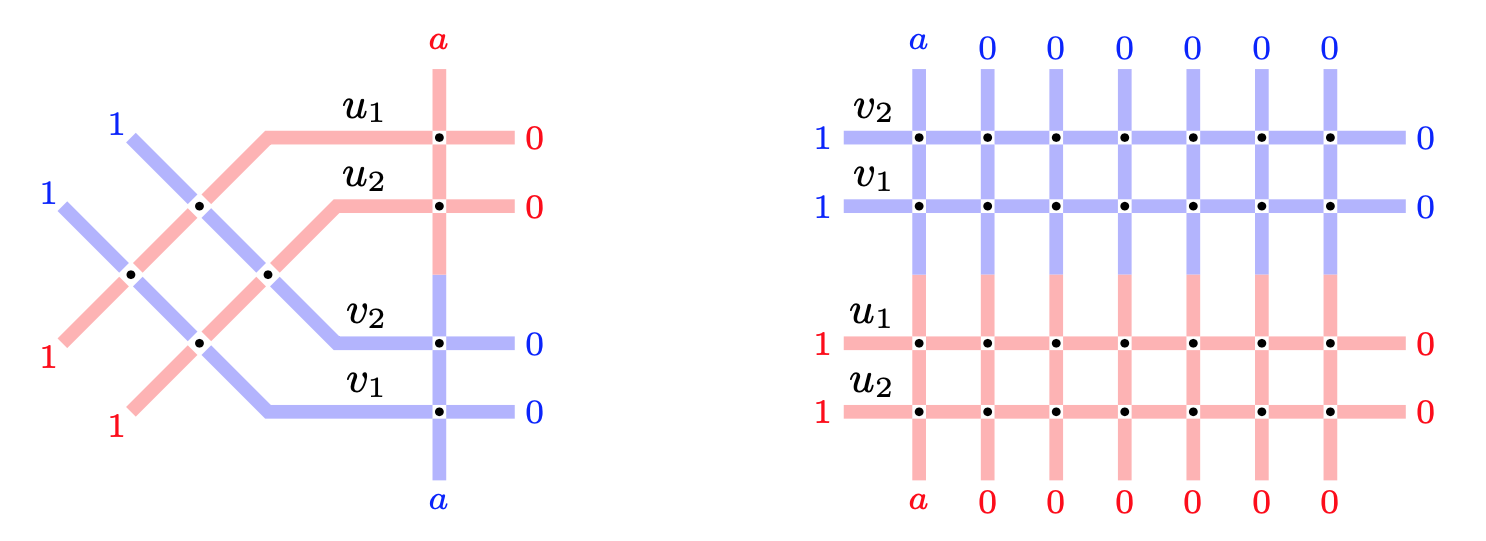# Refined Cauchy identity for spin Hall-Littlewood symmetric rational functions

### 2020/07/20

arXiv:2007.10886 [math.CO]

PDF

Fully inhomogeneous spin Hall-Littlewood symmetric rational functions $\mathsf{F}_\lambda$ arise in the context of $\mathfrak{sl}(2)$ higher spin six vertex models, and are multiparameter deformations of the classical Hall-Littlewood symmetric polynomials. We obtain a refined Cauchy identity expressing a weighted sum of the product of two $\mathsf{F}_\lambda$’s as a determinant. The determinant is of Izergin-Korepin type: it is the partition function of the six vertex model with suitably decorated domain wall boundary conditions. The proof of equality of two partition functions is based on the Yang-Baxter equation.

We rewrite our Izergin-Korepin type determinant in a different form which includes one of the sets of variables in a completely symmetric way. This determinantal identity might be of independent interest, and also allows to directly link the spin Hall-Littlewood rational functions with (the Hall-Littlewood particular case of) the interpolation Macdonald polynomials. In a different direction, a Schur expansion of our Izergin-Korepin type determinant yields a deformation of Schur symmetric polynomials.

In the spin-$\frac12$ specialization, our refined Cauchy identity leads to a summation identity for eigenfunctions of the ASEP (Asymmetric Simple Exclusion Process), a celebrated stochastic interacting particle system in the Kardar-Parisi-Zhang universality class. This produces explicit integral formulas for certain multitime probabilities in ASEP.Two lattices with equal partition functions. This leads to a refined Cauchy identity